# Test: Electric Field (NCERT)

## 15 Questions MCQ Test Topic-wise MCQ Tests for NEET | Test: Electric Field (NCERT)

Description
Attempt Test: Electric Field (NCERT) | 15 questions in 15 minutes | Mock test for NEET preparation | Free important questions MCQ to study Topic-wise MCQ Tests for NEET for NEET Exam | Download free PDF with solutions
QUESTION: 1

### The force per unit charge is known as

Solution:

The force per unit charge is known as: E= q/F ​
This formula f is hold for electric field.

QUESTION: 2

### Electrical as well as gravitational affects acan be thought to be caused by fields. Which the following is true of an electrical or gravitational field?

Solution:

In physics, concept of field is a model used to explain the influence that a massive body or charged particle extends into the space around itself, producing a force on another massive body or charged body placed in space. Thus, concept of field is used to explain gravitational and electrostatic phenomena.

QUESTION: 3

### The electric field at a point is

Solution:

Electric field at a point is continuous if there is no charge at that point. And the field is discontinuous if there is charge at that point. So both options (b) and (c) are correct.

QUESTION: 4

The dimensional formula of electric intensity is

Solution: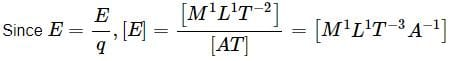QUESTION: 5

If the charge on an object is doubled then electric field becomes

Solution:

As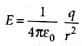If q ' = 2q, then E' =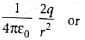E' = 2E
So electric field is doubled.

QUESTION: 6

A force of 2.25 N acts on a charge of 15 x 10-4 C. The intensity of electric field at that point is

Solution: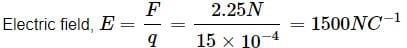QUESTION: 7

A conducting sphere of radius 10 cm has unknown charge. If the electric field at a distance 20 cm from the centre of the sphere is 1.2 x 103N C-1 and points radially inwards. The net charge on the sphere is

Solution:

Here, distance of point from the centre of the sphere, r = 20 cm = 0.2m
Electric field, E =  -1.2 x 103 N C-1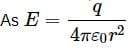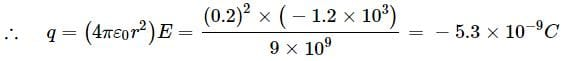QUESTION: 8

A particle of mass 10-3kg and charge 5μC is thrown at a speed of 20m s-1 against a uniform electric field of strength 2 x 105 N C-1. The distance travelled by particle before coming to rest is

Solution:

F = qE = 5 x 10-6 x 2 x 105 = 1 N
Since, the particle is thrown against the field
∴ a = -F/m = -(1/10-3) = 103ms-2
As v2 - u2 = 2as
∴ 02 - (20)2 = 2 x (-103) x s or s = 0.2 m

QUESTION: 9

An electron initially at rest falls a distance of 1.5 cm in a uniform electric field of magnitude 2 x 104N/C. The time taken by the electron to fall this distance is

Solution:

In figure the field is upward. So the negatively charged electron experiences a downward force.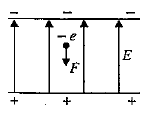∴ The acceleration of electron is
ac = eE/me
The time required by the electron to fall through a distance h is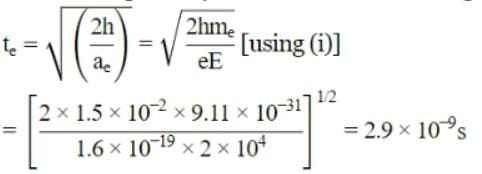QUESTION: 10

The electric field that can balance a charged particle of mass 3.2 x 10-27 kg is (Given that the charge on the particle is 1.6 x 10-19 C)

Solution:

Here, m = 3.2 x 10-27 kg, e = 1.6 x 10-19 C, g = 9.8 m s-2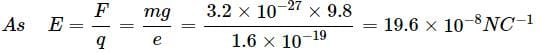QUESTION: 11

An oil drop of 10 excess electrons is held stationary under a constant electric field of 3.65 x 104 N C-1 in Millikan's oil drop experiment. The density of oil is 1.26 g cm-3. Radius of the oil drop is
(Take, g = 9.8 m s-2, e = 1.6 x 10-19 C)

Solution:

Here, n = 10, E = 3.65 x 10N C-1
ρoil = 1.26gcm-3 = 1.26 x 103 kg m-3
As the droplet is stationary weight of droplet = force due to electric field
or (4/3)πr3ρg = neE ∴ r3 = 3neE/4πρg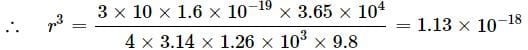or r = (1.13 x 10-18)1/3= 1.04 x 10-6 m

QUESTION: 12

Five equal charges each of value q are placed at the corners of a regular pentagon of side a. The electric field at the centre of the pentagon is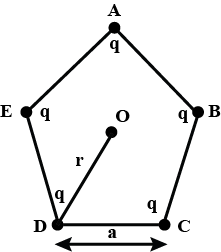Solution:

The electric field at the centre of pentagon would be zero.

QUESTION: 13

Five equal charges each of value q are placed at the corners of a regular pentagon of side a. What will be the electric field at centre O, if the charge from one of the corners (say A) is removed?Solution:

When a charge q from corner A is removed, electric field at O is E1 = (q/4πε0r2) along OA

QUESTION: 14

In question number 45, what will be the electric field at O if the charge q at A is replaced by -q?

Solution:

If the charge q at A is replaced by -q, it is equivalent to adding charge -2q at A. Therefore, electric field at O, E2 = (2q/4πε0r2) along OA.

QUESTION: 15

The tracks of three charged particles in a uniform electrostatic field as shown in the figure. Which particle has the highest charge to mass ratio?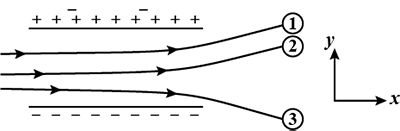Solution:

Particles A and B have negative charges because they are being deflected towards the positive plate of the electrostatic field. Particle C has positive charge because it is being deflected towards the negative plate.
∴ Deflection of charged particle in time t in y-direction
h = 0 x t + (1/2)at2 = (1/2)(qE/m)t2 i.e., h ∝ q/m
As the particle C suffers maximum deflection in y-direction, so it has highest charge to mass q/m ratio.Use Code STAYHOME200 and get INR 200 additional OFF Use Coupon Code

### How to Prepare for NEET

Read our guide to prepare for NEET which is created by Toppers & the best Teachers